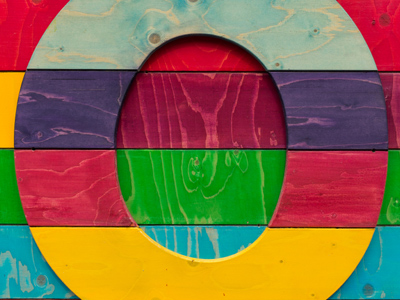All the numbers in the 10 times table end with a 0.

# Ten Times Table

This Math quiz is called 'Ten Times Table' and it has been written by teachers to help you if you are studying the subject at elementary school. Playing educational quizzes is an enjoyable way to learn if you are in the 3rd, 4th or 5th grade - aged 8 to 11.

It costs only \$12.50 per month to play this quiz and over 3,500 others that help you with your school work. You can subscribe on the page at Join Us

In elementary school Math and Numeracy children will be exposed to the times tables as early as possible. The 1, 2, 5 and 10 times tables are taught first before the 3, 4, 6, 7, 8 and 9 times tables follow.

This quiz will test children's familiarity with their ten times table, which will help them in studying math.

Do you know your ten times table? Let's find out!

1.
10 x 0 =
100
1
10
0
Even 10 becomes 0 when it is multiplied by 0
2.
10 x 5 =
60
55
45
50
To times a number by 10 just add a 0 to the end of it
3.
10 x 10 =
90
100
95
99
100 is 10 lots of 10
4.
3 x 10 =
35
40
30
32
5.
6 x 10 =
60
70
72
50
6.
10 x 9 =
99
95
90
93
All numbers in the ten times table end with a 0
7.
10 x 1 =
11
1
0
10
If a number is multiplied by 1 it will stay the same - even the number 10!
8.
7 x 10 =
60
70
80
75
9.
2 x 10 =
12
15
20
5
10.
4 x 10 =
40
30
50
35
Author:  Graeme Haw